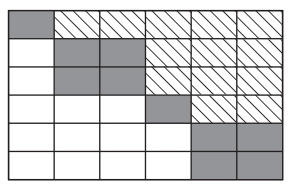# ordqz

Reorder eigenvalues in QZ factorization

## Syntax

``[AAS,BBS,QS,ZS] = ordqz(AA,BB,Q,Z,select)``
``[AAS,BBS,QS,ZS] = ordqz(AA,BB,Q,Z,keyword)``
``[AAS,BBS,QS,ZS] = ordqz(AA,BB,Q,Z,clusters)``

## Description

````[AAS,BBS,QS,ZS] = ordqz(AA,BB,Q,Z,select)` reorders the QZ factorization `Q*A*Z = AA` and ```Q*B*Z = BB``` produced by `[AA,BB,Q,Z] = qz(A,B)` and returns the reordered matrix pair `(AAS,BBS)` along with orthogonal matrices `(QS,ZS)`, such that `QS*A*ZS = AAS` and `QS*B*ZS = BBS`.In this reordering, the selected cluster of eigenvalues appears in the leading (upper left) diagonal blocks of the quasitriangular pair `(AAS,BBS)`. The leading columns of `ZS` span the corresponding invariant subspace. The logical vector `select` specifies the selected cluster as `e(select)`, where `e = ordeig(AA,BB)`.```

example

````[AAS,BBS,QS,ZS] = ordqz(AA,BB,Q,Z,keyword)` sets the selected cluster to include all eigenvalues in the region specified by `keyword`.```
````[AAS,BBS,QS,ZS] = ordqz(AA,BB,Q,Z,clusters)` reorders multiple clusters simultaneously. `ordqz` sorts the specified clusters in descending order along the diagonal of `(AAS,BBS)`, with the cluster of highest index appearing in the upper left corner.```

## Examples

collapse all

Compute the QZ factorization of a pair of matrices, and then reorder the factors according to a specified ordering of the eigenvalues.

Find the QZ factorization, or generalized Schur decomposition, of a pair of matrices `A` and `B`. This decomposition results in the factors $\mathrm{AA}=\mathrm{QAZ}$ and $\mathrm{BB}=\mathrm{QBZ}$.

```A = magic(5); B = hilb(5); [AA,BB,Q,Z] = qz(A,B)```
```AA = 5×5 14.5272 -2.3517 8.5757 -0.2350 -1.4432 0 -19.7471 2.1824 4.5417 7.2059 0 0 -17.9538 8.9292 -9.6961 0 0 0 30.3449 -47.9191 0 0 0 0 32.4399 ```
```BB = 5×5 0.0000 0.0005 0.0018 0.0465 0.2304 0 0.0008 0.0199 0.1662 0.7320 0 0 0.0210 0.1006 -0.1341 0 0 0 0.0623 -1.1380 0 0 0 0 0.7434 ```
```Q = 5×5 -0.1743 -0.1099 -0.0789 -0.4690 0.8552 -0.7567 -0.1151 -0.0846 0.6172 0.1617 -0.4010 0.6782 0.5478 -0.2664 -0.0901 0.4178 -0.0297 0.6473 0.4883 0.4089 -0.2484 -0.7168 0.5173 -0.2995 -0.2593 ```
```Z = 5×5 0.0057 -0.0424 -0.2914 -0.5860 -0.7549 -0.1125 0.4109 0.7635 0.1734 -0.4533 0.4995 -0.6746 0.1486 0.4053 -0.3303 -0.7694 -0.2140 -0.2614 0.4749 -0.2616 0.3818 0.5731 -0.4917 0.4866 -0.2173 ```

Since `AA` and `BB` are triangular, use `ordeig` to extract the eigenvalues from the diagonal blocks of `AA` and `BB`.

`e = ordeig(AA,BB)`
```e = 5×1 106 × 2.8871 -0.0257 -0.0009 0.0005 0.0000 ```

Separate the eigenvalues into clusters, with the real positive eigenvalues ($\mathit{e}>0$) forming the leading cluster. Reorder the matrices `AA`, `BB`, `Q`, and `Z` according to this ordering of the eigenvalues.

`[AAS,BBS,QS,ZS] = ordqz(AA,BB,Q,Z,'rhp')`
```AAS = 5×5 14.5272 -1.2849 1.0391 -7.6821 4.4119 0 21.7128 -19.1784 -1.8380 9.1187 0 0 60.3083 8.4452 -6.4304 0 0 0 -18.2081 3.3783 0 0 0 0 -14.6375 ```
```BBS = 5×5 0.0000 0.0114 0.1908 0.1119 0.0788 0 0.0446 0.0377 0.1107 0.1978 0 0 1.3820 0.6325 0.2807 0 0 0 0.0007 -0.0137 0 0 0 0 0.0171 ```
```QS = 5×5 -0.1743 -0.1099 -0.0789 -0.4690 0.8552 -0.6353 0.1853 0.4099 0.5765 0.2483 -0.7034 -0.4518 -0.3456 -0.2295 -0.3591 0.1415 -0.2036 -0.7054 0.6065 0.2703 -0.2263 0.8414 -0.4568 -0.1647 -0.0705 ```
```ZS = 5×5 0.0057 -0.0088 -0.5288 -0.3591 -0.7690 -0.1125 -0.6095 -0.3858 -0.4737 0.4926 0.4995 0.6478 -0.2711 -0.3644 0.3529 -0.7694 0.4176 -0.4090 0.1750 0.1890 0.3818 -0.1855 -0.5752 0.6952 0.0758 ```

Examine the new eigenvalue order.

`E2 = ordeig(AAS,BBS)`
```E2 = 5×1 106 × 2.8871 0.0005 0.0000 -0.0257 -0.0009 ```

## Input Arguments

collapse all

Matrix factors, specified as the matrices returned by ```[AA,BB,Q,Z] = qz(A,B)```. These matrices satisfy ```Q*A*Z = AA``` and `Q*B*Z = BB`. For complex matrices, `AA` and `BB` are triangular.

If `AA` and `BB` do not form a valid QZ decomposition, then `ordqz` does not produce an error and returns incorrect results.

Data Types: `single` | `double`
Complex Number Support: Yes

Unitary matrices, specified as the matrices returned by `[AA,BB,Q,Z] = qz(A,B)`. These matrices satisfy `Q*A*Z = AA` and `Q*B*Z = BB`.

Data Types: `single` | `double`
Complex Number Support: Yes

Cluster selector, specified as a logical vector with length equal to the number of generalized eigenvalues. The generalized eigenvalues appear along the diagonal of `AA-λ*BB`.

Data Types: `logical`

Eigenvalue region keyword, specified as one of the options in this table.

Option

Selected Region

(`e = ordeig(AA,BB)`)

`'lhp'`

Left-half plane (```real(e) < 0```)

`'rhp'`

Right-half plane (```real(e) >= 0```)

`'udi'`

Interior of unit disk (```abs(e) < 1```)

`'udo'`

Exterior of unit disk (```abs(e) >= 1```)

Cluster indices, specified as a vector of positive integers with length equal to the number of eigenvalues. `clusters` assigns each eigenvalue returned by `e = ordeig(AA,BB)` to a different cluster. All eigenvalues with the same index value in `clusters` form one cluster.

Example: `ordqz(AA,BB,Q,Z,[1 1 2 3 3])` groups five eigenvalues into three clusters.

Data Types: `single` | `double`

## Output Arguments

collapse all

Reordered matrices, returned as matrices that satisfy ```QS*A*ZS = AAS``` and `QS*B*ZS = BBS`.

`QS` and `ZS` are unitary, while `AAS` is quasitriangular and `BBS` is triangular.

collapse all

### Quasitriangular

An upper quasitriangular matrix can result from the Schur decomposition or generalized Schur (QZ) decomposition of real matrices. These matrices are block upper triangular, with 1-by-1 and 2-by-2 blocks along the diagonal.The eigenvalues of these diagonal blocks are also the eigenvalues of the matrix. The 1-by-1 blocks correspond to real eigenvalues, and the 2-by-2 blocks correspond to complex conjugate eigenvalue pairs.

## Tips

• If `AA` has complex conjugate pairs (nonzero elements on the subdiagonal), then you should move the pair to the same cluster. Otherwise, `ordqz` acts to keep the pair together:

• If `select` is not the same for two eigenvalues in a conjugate pair, then `ordqz` treats both as selected.

• If `clusters` is not the same for two eigenvalues in a conjugate pair, then `ordqz` treats both as part of the cluster with larger index.